# Differential of a function, finding the differential

## The concept of differential

Definition: the Differential of the function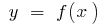at pointis defined as a product of the derivativeat this point, that is,the increase of the argument(denoted by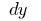or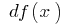— read "teh y")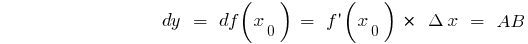For any point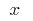:if youhave, then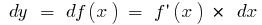## Table of differentials of elementary functions:

1.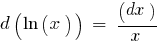2.3.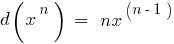4.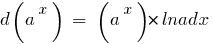5.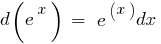6.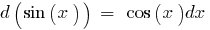7.8.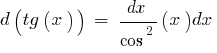9.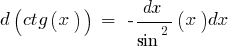An example of finding a differential in mathematics: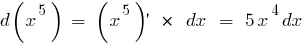The differential is composed of functions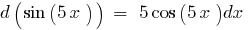## The main property of differential

Differential of a function-the main linear (i.e. proportional) part of the increment function

## The finding of the differential. The geometrical meaning of the differential.

The rules for finding the differential remains the same as that for finding the derivative, you only need to multiply the derivative on DX.If in formula(when there are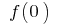and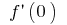), then for small. Let us denote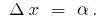Then for small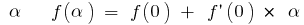For example:

a)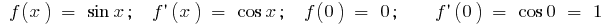, that is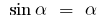(for small) ;

b)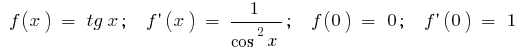, that is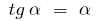(for small)

An example of calculating the differentials For numerical calculations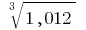we take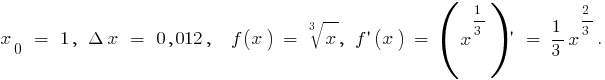Then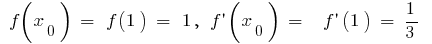the formula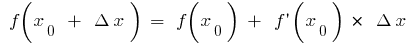gives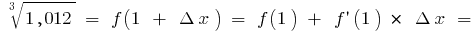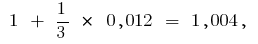that isTags:
Chapter:
Versions in other languages:
Share with friends: